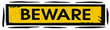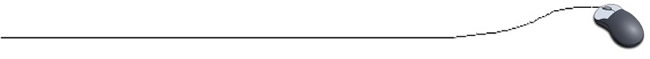Math Operators+  - * / % Addition  Subtraction Multiplication Division Modulus (also called remainder) Notice:  no exponent!  While there is no exponentiation symbol in Java, there is a Math.pow( ) method which will be discussed later.

 The MODULUS Operator % The modulus operator finds the modulus of its first operand with respect to the second.  That is, it produces the remainder of dividing the first value by the second value.  For example: 22 % 6 = 4 because 22 / 6 = 3 with a remainder of 4 Most of our work with the modulus operator will deal with integer values. The modulus, however, may also be applied to doubles.  6.3 % 4.8 = 1.5 because 6.3 / 4.8 = 1 with a remainder of 1.5

Confusing DIVISIONSBe careful when performing integer division.  When dividing an integer by an integer, the Java answer will be an integer (not rounded). But mathematically, we know that an integer divided by an integer may not always be an integer (9 / 4 = 2.5), as integers are not closed under division.

Compare these divisions:  (5 is an integer while 5.0 is a double)

 Integer division 8 / 5 = 1 Double division 8.0 / 5.0 = 1.6 Mixed division 8.0 / 5 = 1.6

When an operation involves two types (as the mixed division shown above), the smaller type is converted to the larger type.  In the case above, the integer 5 was converted to a double type before the division occurred.

Mixed Mode Operations
Consider these examples:

 int a; double b, c; a = 3; b = 5.1; c= a + b; When adding an int to a double, the int is converted to a double for the purpose of adding.  The memory space retains the int. The location of the sum, c, must be a double. int a, b; double c; b = 21; a = 5; c = b/a; Integer division takes place and gives an answer of 4.  This answer is stored as a double 4.0. But what if we wanted the correct division answer ... c = ( double) b/a; c= 21.0 / 5 c = 4.2 It is possible to force the type you want by type casting.   Be careful to force the double to either the numerator or denominator, not both. Note: c = ( double) (b/a); would give an answer 4.0 since integer division would be done first.

Order of Operations
The normal rules you learned in mathematics for order of operations also apply to programming.  The answer to   2 + 7 * 3  is  23   (not 27).   In math you learned that the use of PEMDAS (Please Excuse My Dear Aunt Sally) was helpful for determining order of operations.